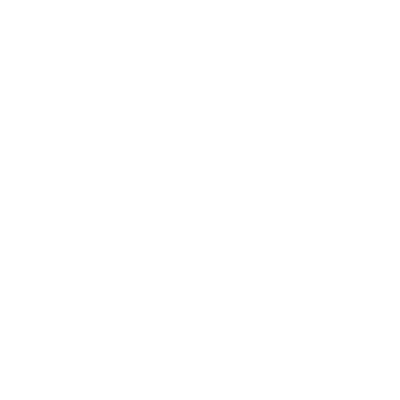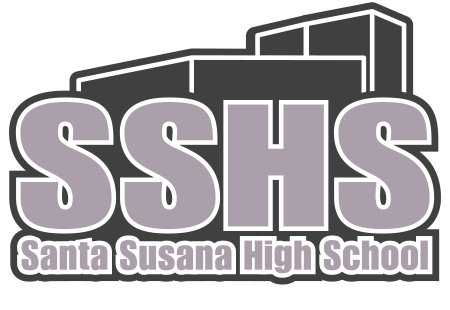Mathematics

# SANTA SUSANA HIGH SCHOOLSanta Susana is the only high school in Simi Valley which offers Honor Calculus C  to their students. This opportunity allows high achieving students to further their mathematical knowledge in preparation to a 4 year University. Many Students that have completed our Mathematics pathway has been accepted into prestigious institutions such as UC Los Angeles, UC San Diego, UC Santa Barbara,  UC Berkeley, USC, Cal Poly SLO, Cooper Union NY, Carnegie Mellon University, Duke University and many other universities. In addition multiple students have been accepted into Berkeley’s Honor Engineering Program with full ride scholarship.

# The Academy of Mathematics at Santa Susana's students, ranks among the top scoring on state test in Mathematics.

The Academy of Accelerated Academics at Santa Susana High School is one of the top programs in Ventura County. Students at SSHS are among to top scoring on state test in Mathematics. This is because of the rigorous program that Santa Susana provides for each of their students. Students choose math classes that will benefit their future goals. For example if a student is interested in pursuing a STEM field occupation, they have the opportunity to take 3 years of calculus, which other high schools in the area do not offer. Students may pursue the option of taking AP Statistics after completing Algebra 2. The Mathematics Pathway includes the basic math courses, but students are able to take accelerated math courses such as Algebra 2 / Trigonometry and then go into calculus their Sophomore or Junior year.  These Mathematical courses will develop critical thinking skills necessary for success in the future.

Honors Calculus C (10 Units)
This course covers the study of vector calculus in three dimensional space, and includes topics such as dot and cross products, using spherical and cylindrical coordinates to evaluate and explore applications of integrals of several variables, partial derivatives and their applications, gradients, Lagrange multipliers, vector calculus with line and surface integrals, Green’s, Stokes’ and Divergence Theorems and applications, and an introduction to second - order differential equations. This course will also include a review of Trigonometry, Calculus AB and BC topics.

AP Calculus BC (10 Units)
Calculus BC is a full-year course in the calculus of functions of a single variable. It includes all the topics covered in Calculus AB plus additional topics.  Additional topics include finding the solution to logistic differential equations, integration using trigonometric functions, parametric and polar functions, finding a polynomial representation of a function (Taylor or Maclaurin series), and finding the interval of convergence of a series.

AP Calculus AB (10 Units)
This Advanced Placement course is intended for students who have a thorough knowledge of college preparatory pre-calculus mathematics including Algebra, Geometry and Trigonometry. A score of 3, 4 or 5 on the examination will enable the student to obtain 3 semester units of college credits in mathematics. Graphing calculators and computers will be used throughout the course.  Students need to become proficient in the uses of the graphing calculator in preparation for the Advanced Placement Exam in order to solve problems involving more complex expressions.  Topics covered in the course include limits, differential calculus and applications, integral calculus and applications, and solutions to elementary differential equations.

AP Statistics (10 Units)
The statistics presented are primarily descriptive. Newspapers, magazines and televised programs will be used to introduce and discuss the following topics: table graphs (circle, bar, line and histograms); plots (scatter, stem-and-leaf and box-and-whiskers); measures of central tendency; measures of deviation; quartiles, percentiles, variance, Z-scores and others. Students will become familiar with statistics calculators and use software packages.

CP Statistics (10 Units)
The course is intended for students who have a strong knowledge of college preparatory mathematics including algebra, geometry, and second-year algebra. Topics covered include: Exploring Data: Observing patterns and departures from patterns. Planning a Study: Deciding what and how to measure. Anticipating Patterns in Advance: Producing models using probability and simulation. Statistical Inference: Confirming models.

Pre-Calculus (10 Units)
Pre-Calculus is a full-year course that blends together all of the pre-calculus concepts and skills that must be mastered prior to the enrollment in a college level calculus course. A functional approach integrating as many concepts as possible will be used throughout the course. Implementation of current technology, such as graphing calculators and computer-assisted instruction, will be used in the development of the skills and concepts.

Algebra II and Trigonometry (10 Units)
This course is offered for academically exceptional students who intend on enrolling in AP Calculus in the following school year. Students entering the course will be expected to demonstrate creativity, imagination and have the ability to apply Algebra in various situations. The course will emphasize the fundamentals of Algebra and will develop the trigonometric functions through the use of both right triangle and the unit circle. Implementation of current technology, such as graphing calculators and computer-assisted instruction, will be used in the development of the skills and concepts. Students will be expected to apply concepts from class to real-world situations to extend their learning.

Algebra II (10 Units)
This course expands the mathematical content of Algebra I and Geometry.  Review of those concepts are integrated throughout the course.  Emphasis will be placed on abstract thinking skills, functions and the algebraic solution of problems in various content areas.  These areas emphasized are  quadratic, rational, polynomial, radical,  logarithmic,  exponential and trigonometric functions.  In addition, probability, sequences and series are topics for further exploration.

Geometry (10 Units)
Geometry is a formal development of the geometric skills and concepts necessary for students who will take Algebra II and/or other advanced college preparatory courses. In particular, the course will provide for the consistent use of algebra throughout the content. Students will be expected to use a full range of problem solving skills which will help them develop their critical thinking skills. Trigonometry will also be introduced as well during this course.  Throughout the year students will be asked to apply concepts being taught to real-life application as well as justify their reasoning and thinking.

Business Algebra 1  is designed to develop mathematical skills that are taught in a regular Algebra 1 class and then apply that knowledge to real-life application using authentic learning.  Students will learn the fundamentals of mathematical modeling,  banking, statistics, and probability.

Algebra I (10 Units)
Algebra is the language through which most mathematics is communicated. Through the study of algebra, students develop understanding of mathematical functions and concepts used in a wide variety of problem-solving situations. In Algebra 1 students gain the tools that they will need to be successful in higher level math courses.

Certification Requirements
Recognition: 3.0 GPA in all Math classes as well as the completion PreCalculus or AP Statistics.

Honors: 3.5 GPA in all Math classes as well the completion of Calculus AB or AP Statistics

High Honors: 4.0 GPA in all Math Classes as well as the Completion of AP Calculus BC + One of the following: AP Physics, AP Statistics,  AP Computer Science, AP Chemistry, or Calculus C Honors

Courses and Course #’s
Algebra I (10 units) (Y) H5161 / H5162
Geometry (10 units) (Y) #H5169 / H5170
Algebra II (10 units) (Y) #H5179 / H5180
Algebra II and Trigonometry (10 units) (Y) #H8049 / H8050CP Statistics (10 units) (Y) #H8275 / H8276
AP Statistics (10 units) (Y) #H5667 / H5668
Pre-Calculus (10 units) (Y) #H5173 / H5174
AP Calculus AB (10 units) (Y) #H5493 / H5494
AP Calculus BC (10 units) (Y) #H7399 / H7400
Honors Calculus C (10 units) (Y) #H8329 / H8330Santa Susana High School
3570 E. Cochran St.
Simi Valley, CA 93063
805.520.6800
www.santasusana.org